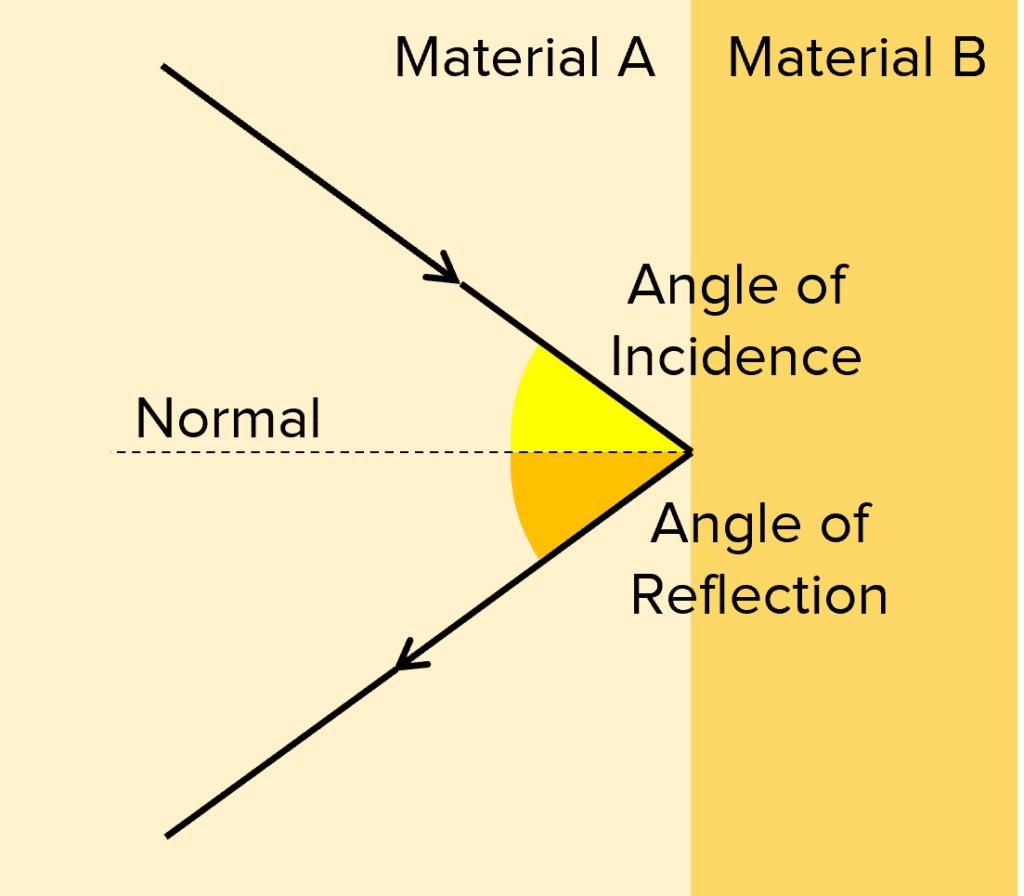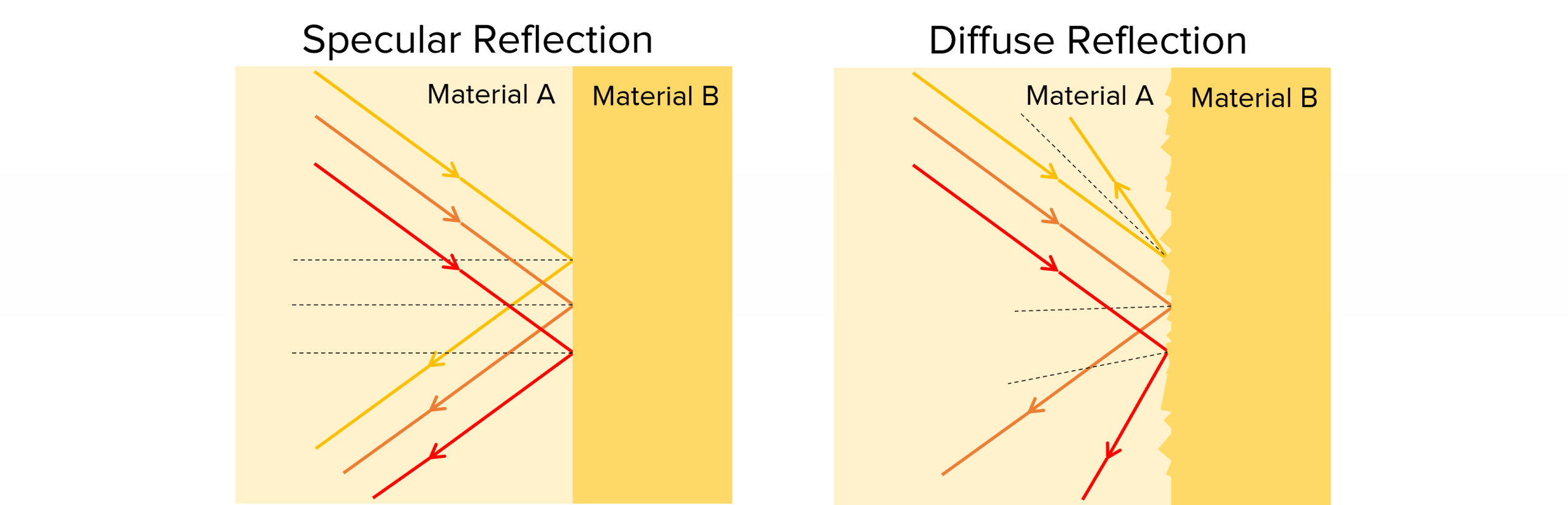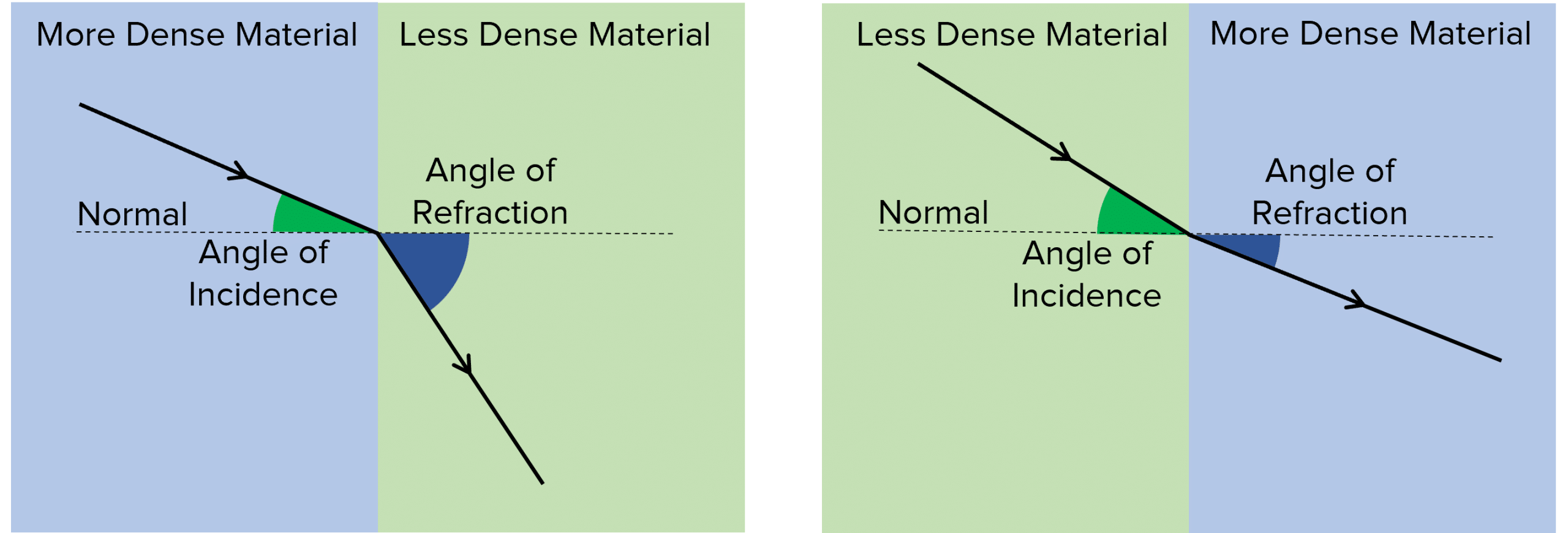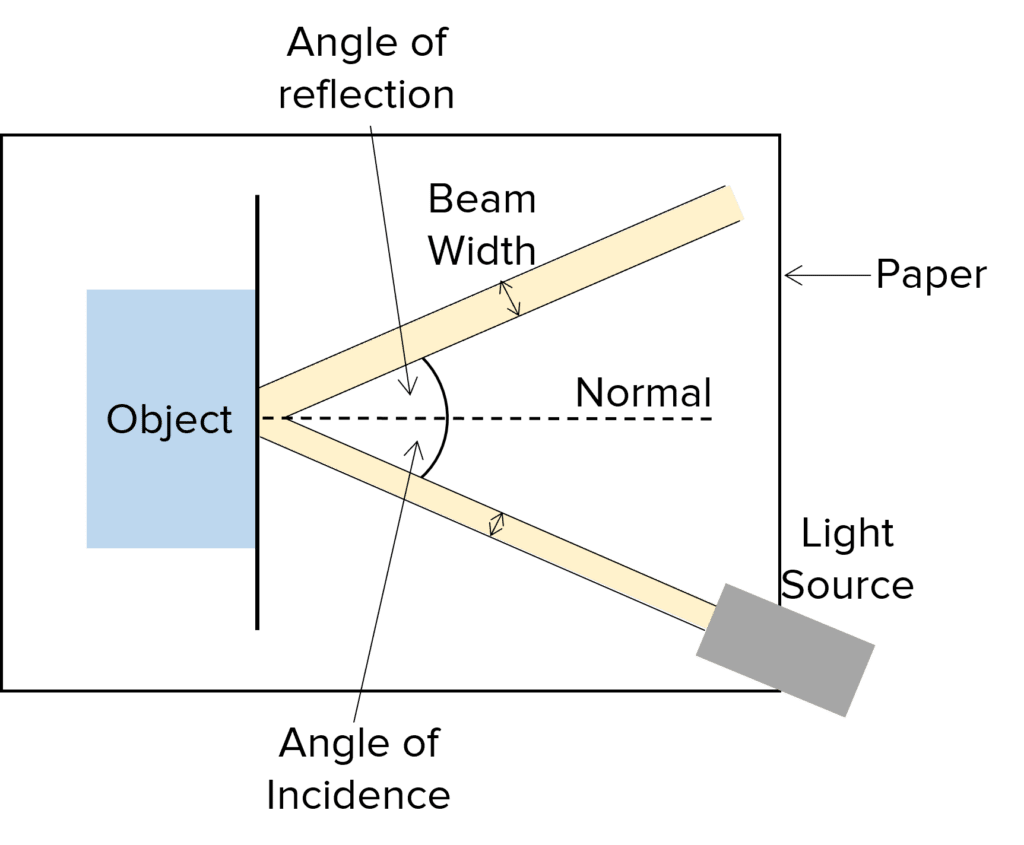# Reflection and Refraction

GCSEAQAPhysics FoundationPhysics Higher

## Reflection and Refraction

At boundaries between materials, waves can refract (bend), reflect or be absorbed. This depends on the properties of the materials and the wavelength of the waves.

## ReflectionReflection is where the wave bounces off a material boundary. There is one rule to remember for reflection:

$\text{angle of incidence}=\text{angle of reflection}$

The angle of incidence is the angle between the incoming wave and the normal to the material boundary.

The angle of reflection is the angle between the reflected wave and the normal to the material boundary.

The normal is a line at right angles with the boundary.

The diagram shows reflection on a wave diagram. Wave diagrams are used to show the path of waves. Straight lines are used to represent the path of the wave and arrows represent the direction of travel of the wave. The normal is drawn as a dotted line

GCSEPhysics FoundationPhysics HigherAQA

## Specular and Diffuse Reflection

Reflection can be either specular or diffuse

Specular reflection is when you get a clear reflection, where all of the waves bounce off the boundary at the same angle. As a result, the surface appears shiny. This occurs when waves are reflected on smooth surfaces such as light on a mirror.

Diffuse reflection is when waves are reflected off a rough surface, which causes waves to be reflected by many different angles due to the bumps on the surface. The rule of $\text{angle of incidence}=\text{angle of reflection}$ still applies, but because of the rough surface, each wave has a different angle of incidence. Hence, each wave also has a different angle of reflection. This causes the surface to appear matte and you don’t get a clear reflection.GCSEPhysics FoundationPhysics HigherAQA

## Refraction

Refraction is when waves change direction as they cross a boundary.

Waves are only refracted if the wave meets the boundary at an angle. The amount the wave changes direction depends on the difference on the materials it is travelling between.  This is because waves travel different speeds in different materials.

If the wave speeds up in the new material, its path will bend away from the normal.

If the wave slows down in the new material, its path will bend toward to the normal

Usually, waves travel slower in denser materials. A notable exception to this rule is sound waves, which travel faster in denser materials.GCSEPhysics FoundationPhysics HigherAQA

## Required Practical

Investigation into Reflection Off Different Materials

You can perform the following experiment to show that $\text{angle of incidence}=\text{angle of reflection}$ as well as finding out which type of reflection (specular or diffuse) occurs on different materials.

Doing the experiment1. Use a ruler to draw a straight line on a piece of paper.
2. Place an object on top of the piece of paper so one of its edges lines up with the straight line you have drawn.
3. Shine a ray of light at the object and trace the outline of the incoming and reflected beam on the piece of paper.
4. Draw the normal to the straight line at the point that the light hit the object.
5. Measure the angle of incidence and angle of reflection of the object using a protractor.
6. Measure the width of the incident and reflected beams.
7. Repeat for a range of objects, made from different materials.

You should find that $\text{angle of incidence}=\text{angle of reflection}$. If the beam is wider after it has been reflected, this means that diffuse reflection has occurred, as the light has been scattered in different directions.

GCSEPhysics FoundationPhysics HigherAQA

## Reflection and Refraction Example Questions

$\text{angle of incidence} = \text{angle of reflection}$

Therefore:

$\text{angle of reflection} = \bold{62 \degree}$Gold Standard Education

If a material has a rough surface, incident light will be reflected at many different angles because the angle of incidence is different at different positions on the rough surface. This is called diffuse reflectionGold Standard Education

Air is less dense than glass and so the electromagnetic wave travels faster in air than glass. So, the wave is refracted at the boundary and the path of the wave bends towards the normal to the boundary.